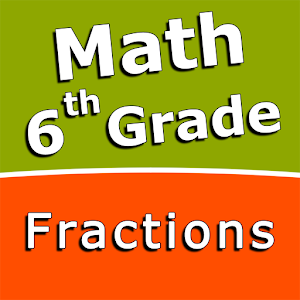Nederlands

We bieden dagelijks GRATIS gelicenseerde Android apps en games aan, die je normaal zou moeten kopen.\$2.99
VERSTREKEN

# Android Giveaway of the Day - Fractions and mixed numbers - 6th grade math

Add fractions and mixed numbers with like denominators.
\$2.99 VERSTREKEN
Waarderingscijfer: 0 (0%) 0 (0%)

Deze giveaway is verlopen. Fractions and mixed numbers - 6th grade math is nu beschikbaar op de normale manier.

Intuitive interface powered by handwriting input and a choice of three fun and engaging mini games in addition to a regular math trainer mode make our app stand out from the crowd of generic math learning apps.

With Fractions and mixed numbers - 6th grade math you can practice and improve the following math skills:
- Write fractions in lowest terms
- Add fractions and mixed numbers with like denominators
- Add fractions and mixed numbers with unlike denominators
- Subtract fractions and mixed numbers with like denominators
- Subtract fractions and mixed numbers with unlike denominators
- Multiply fractions and mixed numbers
- Multiply mixed numbers and whole numbers
- Divide fractions and mixed numbers
- Divide mixed numbers by whole numbers
- Convert fractions and mixed numbers to decimals

Sergey Malugin

Educational

### Versie:

Varies with device

### Grootte:

Varies with device

Everyone

### Compatibiliteit:

Varies with device

## Reactie op Fractions and mixed numbers - 6th grade math

Thank you for voting!